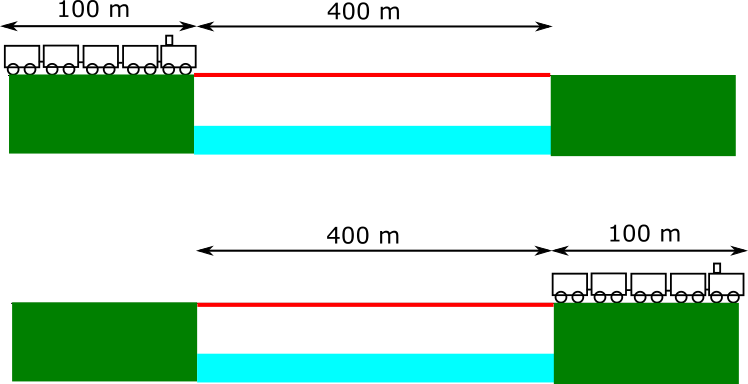#### You may also like### Consecutive Numbers

An investigation involving adding and subtracting sets of consecutive numbers. Lots to find out, lots to explore.### Calendar Capers

Choose any three by three square of dates on a calendar page...### Days and Dates

Investigate how you can work out what day of the week your birthday will be on next year, and the year after...

# Speed over a Bridge

##### Age 11 to 14 ShortChallenge LevelTotal distance travelled to clear the bridge is 500 m (the front of the train ends up 500 m from where it started.)

So the train has travelled 500 m in 30 seconds.

Working up to km/h using multiplication
30 seconds : 500 m
1 minute : 500 m$\times$2 = 1 km
1 hour : 1 km$\times$60 = 60 km

Using division to find the speed
1 hour = 60 minutes = 60$\times$(2$\times$30 seconds) = 120$\times$(30 seconds).

That means there are 120 30-second intervals in an hour. So in an hour, the train would travel this distance 120 times.

120$\times$500 m = 60 000 m = 60 km.

You can find more short problems, arranged by curriculum topic, in our short problems collection.